DNghiem

•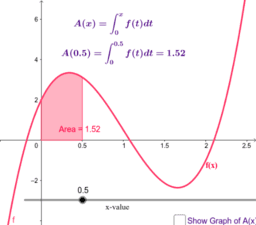Copy of The "Area-Eating Function"

Activity

DNghiem

•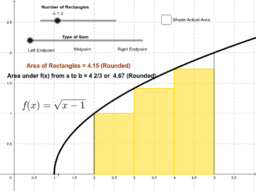Left, Right, and Midpoint Sum

Activity

DNghiem

•Copy of Left, Right, and Midpoint Sum

Activity

DNghiem

•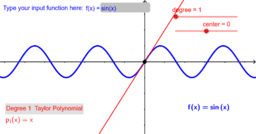Taylor Polynomial Generator

Activity

DNghiem

•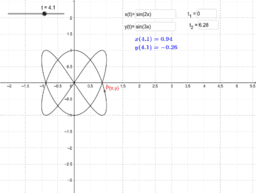Parametric Equation Sketcher

Activity

DNghiem

•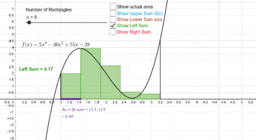Exploring Upper and Lower Riemann Sums

Activity

DNghiem

•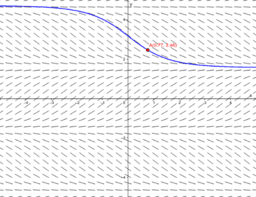Slope Field Generator

Activity

DNghiem

•The "Area-Eating Function"

Activity

DNghiem

•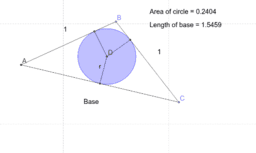Optimized Circle Inscribed in an Isosceles Triangles

Activity

DNghiem

•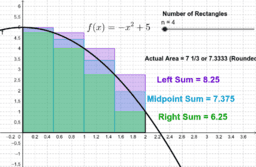Comparing Left, Right, and Midpoint Riemann Sums

Activity

DNghiem

•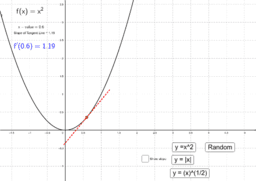Slope of Tangent Lines

Activity

DNghiem

•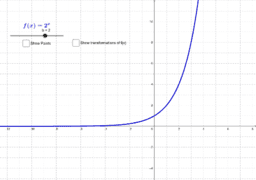Exponential Functions and Transformations

Activity

DNghiem

•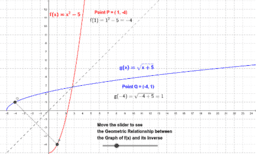The Graph of f(x) vs its Inverse

Activity

DNghiem

•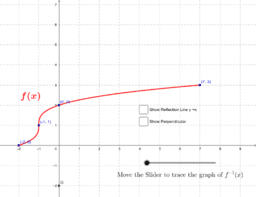Sketching an Inverse Function

Activity

DNghiem

•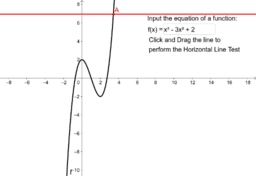Horizontal Line Test

Activity

DNghiem

•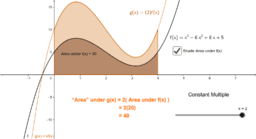Activity

DNghiem